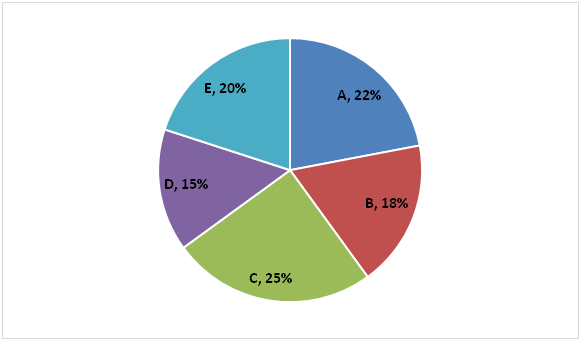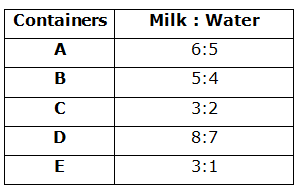# NIACL AO Mains– Quantitative Aptitude Questions Day- 12

Dear Readers, Bank Exam Race for the Year 2019 is already started, To enrich your preparation here we have providing new series of Practice Questions on Quantitative Aptitude – Section. Candidates those who are preparing for NIACL AO Mains 2019 Exams can practice these questions daily and make your preparation effective.

[WpProQuiz 5177]

1) A, B and C started a business by investing Rs. 18000, Rs. 24000 and Rs. 32000 respectively. After one year, A invested 50 % more than the initial investment but C withdraws Rs. 7000. And after one more year, B invested Rs. 6000. Find the total profit at the end of three years, if the share of B is Rs. 97500?

a) Rs. 290000

b) Rs. 275000

c) Rs. 240000

d) Rs. 195000

e) None of these

2) Abinav invested a sum of Rs. 40000 on simple interest at 6 % per annum for 3 years. He invested a same amount on Compound interest at 4 % per annum for 2 years. Find the total interest received by him after a given period?

a) Rs. 9128

b) Rs. 10464

c) Rs. 11572

d) Rs. 9956

e) None of these

3) A shopkeeper sold an item at a discount of 15 % but he gains 25 %. If the marked of the item is Rs. 25000, then find the cost price of the item?

a) Rs. 19000

b) Rs. 18000

c) Rs. 17000

d) Rs. 20000

e) None of these

4) A and B can complete a piece of work in 12 days and 16 days respectively. With the help of C, they can complete the work in 6 days. Find D alone can complete the work in, if the efficiency of D is three times the efficiency of C?

a) 20 days

b) 24 days

c) 28 days

d) 16 days

e) None of these

5) The ratio between the radius to that of the height of a cylinder is 1: 3 and the perimeter of a square is 112 cm. Find the volume of a cylinder, if the radius of the cylinder is equal to one-fourth of the side of the square?

a) 3234 Sq cm

b) 2986 Sq cm

c) 2572 Sq cm

d) 3458 Sq cm

e) None of these

Directions (6 – 10): Study the following information carefully and answer the questions given below:

The following pie chart represents percentage wise distribution of Quantity of mixture of milk and water in five different containers.

Total quantity of mixture in the five containers = 1200 litresThe following table represents ratio of milk and water among them.6) The milkman sold 44 litres of the mixture from container A and added 5 litres of pure milk and 10 litres of water to remaining mixture. Find the difference between quantity of milk and quantity of water in the final mixture of container A.

a) 20 litres

b) 10 litres

c) 25 litres

d) 15 litres

e) None of these

7) If mixtures of container B and container E are mixed in a different container F, find the respective ratio of milk and water in container F.

a) 29:15

b) 23:11

c) 25:13

d) 21:8

e) None of these

8) Find the average of the quantity of milk in container B, container C and container D.

a) 176 litres

b) 132 litres

c) 154 litres

d) 143 litres

e) None of these

9) Quantity of water in which of the given five containers is minimum?

a) A

b) B

c) C

d) D

e) E

10) The milkman added 14 litres pure milk and 6 litres water to container D. He Sold 80 litres of the mixture and again added 4 litres of pure milk and 2 litres of water to the remaining mixture. Find the respective ratio of milk and water in the final mixture.

a) 2:1

b) 3:2

c) 4:3

d) 5:4

e) None of these

The share of A, B and C

= > [18000*1 + 18000*(150/100)*2] : [24000*2 + 30000*1] : [32000*1 + 25000*2]

= > 72000: 78000: 82000

= > 36: 39: 41

The share of B = 97500

39’s = 97500

1’s = 97500/39 = 2500

Total profit = 116’s = Rs. 290000

SI = PNR/100 = (40000*6*3)/100 = Rs. 7200

CI = 40000*(104/100)*(104/100) – 40000

= > 43264 – 40000 = 3264

The total interest received by him after a given period

= > 7200 + 3264 = Rs. 10464

MP*[(100 – D%)/100] = CP*[(100 + P%)/100]

25000*(85/100) = CP*(125/100)

CP = 25000*(85/100)*(100/125) = Rs. 17000

A and B’s one day work = (1/12) + (1/16) = 7/48

C’s one day work = (1/6) – (7/48) = 1/48

C can complete the work in 48 days.

Efficiency ratio = > C: D = 1: 3

Day ratio = > C: D = 3: 1

3’s = 48

1’s = 16

D alone can complete the work in 16 days

The ratio between the radius to that of the height of the cylinder = 1: 3

The perimeter of the square = 112 cm

4a = 112

Side (a) = 112/4 = 28 cm

Radius of the cylinder = (1/4)*28 = 7 cm

Volume of the cylinder = πr2h = (22/7)*7*7*21 = 3234 Sq cm

Direction (6-10) :

Total quantity of mixture in container A = 22/100 x 1200 = 264 litres

Quantity of milk in the initial mixture of container A = 6/11 x 264 = 144 litres

Quantity of water in the initial mixture of container A = 5/11 x 264 = 120 litres

Quantity of milk in the final mixture of container A = 144 – 6/11 x 44 + 5

= 144 – 24 + 5

= 125 litres

Quantity of water in the final mixture of container A = 120 – 5/11 x 44 + 10

= 120 – 20 + 10

= 110 litres

Required difference = 125 – 110 = 15 litres

Quantity of mixture in container B = 18/100 x 1200 = 216 litres.

Quantity of milk in container B = 5/9 x 216 = 120 litres

Quantity of water in container B = 4/9 x 216 = 96 litres

Quantity of mixture in container E = 20/100 x 1200 = 240 litres

Quantity of milk in container E = ¾ x 240 = 180 litres

Quantity of water in container E = ¼ x 240 = 60 litres

Required ratio = (120 + 180): (96 + 60)

= 300: 156

= 25:13

Quantity of milk in container B = 5/9 x 18/100 x 1200 = 120 litres

Quantity of milk in container C = 3/5 x 25/100 x 1200 = 180 litres

Quantity of milk in container D = 8/15 x 15/100 x 1200 = 96 litres

Required average = (120 + 180 + 96)/3

= 396/3

= 132 litres

Quantity of water in container A = 5/11 x 22/100 x 1200 = 120 litres

Quantity of water in container B = 4/9 x 18/100 x 1200 = 96 litres

Quantity of water in container C = 2/5 x 25/100 x 1200 = 120 litres

Quantity of water in container D = 7/15 x 15/100 x 1200 = 84 litres

Quantity of water in container E = ¼ x 20/100 x 1200 = 60 litres (Minimum)

Initial quantity of milk in container D = 8/15 x 15/100 x 1200 = 96 litres

Initial quantity of water in container D = 7/15 x 15/100 x 1200 = 84 litres

Milk = 96 + 14 = 110 litres

Water = 84 + 6 = 90 litres

Milk: Water = 110: 90 = 11:9

Quantity of milk in the final mixture = 110 – 11/20 x 80 + 4

= 110 – 44 + 4

= 70

Quantity of water in the final mixture = 90 – 9/20 x 80 + 2

= 90 – 36 + 2

= 56 litres

Required ratio = 70: 56 = 5: 4